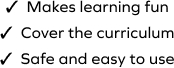# Regroup Once and Subtract Two 4-Digit Numbers

SplashLearn - The complete PreK - Grade 5 Math & ELA Learning Program Built for Your Child
Home > Regroup Once and Subtract Two 4-Digit Numbers
The game is about solving a set of problems of varying difficulty levels and by doing so, your young mathematician will gain fluency in subtraction. The student will regroup once and subtract two 4-digit numbers in this game. Students will drag and drop the items at the correct places to solve the problems.